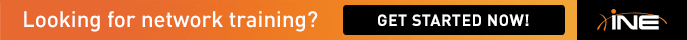Can someone breakdown how in the SG its a /58?  I haven't been able to wrap my head around IPv6 summariztion

R/

Jonathan

• Thanks, from looking at the post I understand the logic now but unsure of the last question of which is correct?  /59 vs /58.  If you break 12 & 25 down in hex  it = C & 19 in binary it = 1100 & 11001.  Based on the last answer of the post I'm not able to correlate the answer from the guys question on which is correct /58 or /59

Jonathan

• let's break hex values in bin:
`2001:141:X:12           000100102001:141:X:25           00100101 `
we apparently have two leading bits the same, thats it we may summarize 'em. Now a little bit of theory: IPv6 address is 128 bit one. Notation break it into eight quartets, each equal to 16 bits(8*16=128). Also notation rules says that we may do not write leading zeros, just omit 'em, but do not forget 'em. In our case we have, for example, I pick one:

2001:141:X:12::/64 or 2001:141:X:0012::/64 , or in binary interested quarted looks like 00000000'00010010

so the least overlapping mask is 16+16+16+10=58
• Had a little difficulty understanding the above explanation, so just to make things a bit more clear for anyone else, we are interested in summarizing the following ipv6 addresses:

2001:141:X:0012::/64

2001:141:X:0025::/64

Taking into consideration only the 4th set of hex values and converting them into binary (each single hex digit is 4 binary digits) we have:

0x0012 = 0000 0000 0001 0010

0x0025 = 0000 0000 0010 0000

As you can see above there are 6 bits that are uncommon, if you subtract this number from the original /64 mask that is applied you are left with your solution summary /58:

2001:141:X::/58 (contiguous 0 blocks are omitted)

HTH

• Thanks, from looking at the post I understand the logic now but unsure of the last question of which is correct?  /59 vs /58.  If you break 12 & 25 down in hex  it = C & 19 in binary it = 1100 & 11001.  Based on the last answer of the post I'm not able to correlate the answer from the guys question on which is correct /58 or /59

Jonathan

Jonathan, you are confusing 12 and 25 with being decimal values as opposed to hex values. It is actually 0x12 and 0x25, see my previous post for the correct conversion from hex to binary. HTH

• Very well explained. I had the binary value's written down but just couldn't figure where the mask value was being calculated. Got it now. Thanks.

• Initially I have the same problem but I think if we go to basics it seems to be easy to understand

It always confuses because they way IPv6 is represented, address is represented by Hexa and prefix is derived from binary.

Normally a byte (8 bits) can represent in Hexa by two hexa bits

Byte = 8 bits range 00000000 to 11111111

Hexa= range 00 – ff  to represent a byte [===this is the key

Mac address format is actually `01:23:45:67:89:ab can also represent as 0123.4567.89ab`

That does't mean all 4 hexas are together, its always 2 hexa bits stay together to represent a byte

Now coming to Ipv6, from above link, I can see they are asking why it is not /59 why it is /58

===========================================

2001:141:1:12::/64
2001:141:1:25::/64

so taking the 4th grouping 12 & 15. I write them in binary like this:
0000 0000 0000 1100 = 12
0000 0000 0001 1001 = 25
------------------------

I come up with 11 bits for the mask.

So in total my mask should be /59... but the solutions guide says /58. Who is correct and why?

===========================================

If I represent them this way answer is easy

00 00  00 00  00 00  11 00 = 12
00 00  00 00  00 01  10 01 = 25

as u can not take 11th bit it self as 11 and 12 bits stay together …. its not always contiguous 0

Regards,

Ananth.

` `

• A little clarification on hexadecimal number representation:

2001:141:1:12::/64 should be expanded to 2001:0141:0001:0012::/64 (or at least the first segment that differs should be expanded to 0012)

2001:141:1:25::/64 should be expanded to 2001:0141:0001:0025::/64 (or 0025)

Remember that each hexadecimal digit represents 4 bits. The 32 hexadecimal digits in the complete (not abbreviated) IPv6 address expand to 128 bits.

0012 in hex will expand to 0000 0000 0001 0010 in binary

0025 in hex will expand to 0000 0000 0010 0101 in binary

The underlined hexadecimal digits represent 48 bits that are the same.

The underlined bits represent the bits of the fourth segment that are the same: 48 + 10 = 58.

===========================================

2001:141:1:12::/64
2001:141:1:25::/64

so taking the 4th grouping 12 & 15. I write them in binary like this:
0000 0000 0000 1100 = 12
0000 0000 0001 1001 = 25
------------------------